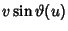## Right Conoid

A Ruled Surface is called a right conoid if it can be generated by moving a straight Line intersecting a fixed straight Line such that the Lines are always Perpendicular (Kreyszig 1991, p. 87). Taking the Perpendicular plane as the-plane and the line to be the x-Axis gives the parametric equations(Gray 1993). Takingandgives the Helicoid.

References

Dixon, R. Mathographics. New York: Dover, p. 20, 1991.

Gray, A. Modern Differential Geometry of Curves and Surfaces. Boca Raton, FL: CRC Press, pp. 351-352, 1993.

Kreyszig, E. Differential Geometry. New York: Dover, 1991.